## Plot Variable confusion...

In my worksheet today my intention was to compare the least squares linear regression for three datasets as indicated, but when I right click on the output as seen in the bottom line to select the plot type, all options state there to be independant variables K and K, where as the output displays only the variable K as I intended, which part of my code is creating this confusion for maple?

Worksheet Specific Investigation Content

 >>>>>(1.1)
 >## Error, (in rtable/Product) invalid arguments while...

 >>>>Hello there,

Here is a set

thanks

## RE: 'fsolve' unable to solve once 'conjugate' oper...

Hello there,

Here is a set of non-linear equations:

 > restart;
 > with(LinearAlgebra):(1)
 >(2)
 > f1n2 := (0.03 + I*0.1515)*Ix[c1] - (0.03 + I*0.1515)*Ix[c2] + 2 * V[at1] - 55*10^3 = 0;(3)
 > f3n4 := (1.6 + I*6.24)*Ix[c1] + (1.12 + I*2.64)*Ix[c2] + V[t] - V[at1] = 0;(4)
 > f5n6 := (1.36 + I*4.44)*Ix[c2] + V[at2] - V[t] = 0;(5)
 > f7n8 := (-1.12 - I*2.64)*Ix[c1] + (-3.92 - I*12.00)*Ix[c2] + V[at2] - V[at1] = 0;(6)
 > f9n10 := V[t] * (Ix[c1] - Ix[c2]) + TrainLoad = 0;(7)
 > polynomials := {f1n2, f3n4, f5n6, f7n8, f9n10};(8)
 > variables := {Ix[c1], Ix[c2], V[at1], V[at2], V[t]};(9)
 > fsolve(polynomials, variables, complex);(10)
 >
 >

The 'fsolve()' command was able to come up with a solution.

Then, when 'f9n10 := V[t] * (Ix[c1] - Ix[c2]) + TrainLoad = 0;' becomes 'f9n10 := V[t] * conjugate(Ix[c1] - Ix[c2]) + TrainLoad = 0;',

the command ('fsolve()') refused to produce a solution.

Would you tell me how to make the 'fsolve()' command work with the 'conjugate()' operator?

Thank you,

In Kwon Park

Hello,

I am one of the software coordinators for CUNY and was wondering how would i obtain access to download Maplesoft for the college community?

## Why is matrix rank evaluating so slowly?...

I have the following program which constructs the multiplication table, CI, for a matrix Lie algebra and evaluates the difference between CI's row dimension and its rank. The code is a little convoluted because "LieTable" formats the entries very strangely and forces incorrect rank values.

The matrix CI is constructed rather quickly (within a few seconds), and everything works well with "small" examples (up to 12 basis elements has evaluated within seconds). However, the example I've included is for a 27-dimensional Lie algebra. As I stated, CI is constructed quickly, even in larger examples, but the rank evaluation (i.e., LinearAlgebra:-Rank(CI)) has never completed for the example I've included. I let it run for about 3 hours before shutting it down.

I have an older Macbook Air which I am using to run these computations. Could this simply be an issue of not enough computing power?

I have attempted to import the matrix CI into Mathematica (to see if it was simply a limitation of Maple), but that's its own headache (reads entries of the matrix incorrectly).

Any recommendations would help. If this is an issue of computing power, I can get access to a more powerful system soon. It doesn't seem that the code itself would cause the issue, since it is not the construction of the matrix which is giving me issues, it is the evaluation of the rank. I am rather naive about Maple (and programming in general) though, so I may be wrong.

Index_and_Contact.mw

## How do I substitute a combination of functions by ...

I want to do the substitution f(t) - ff(t) = epsilon for any variable t in Maple: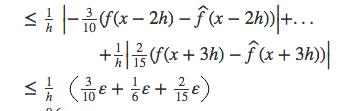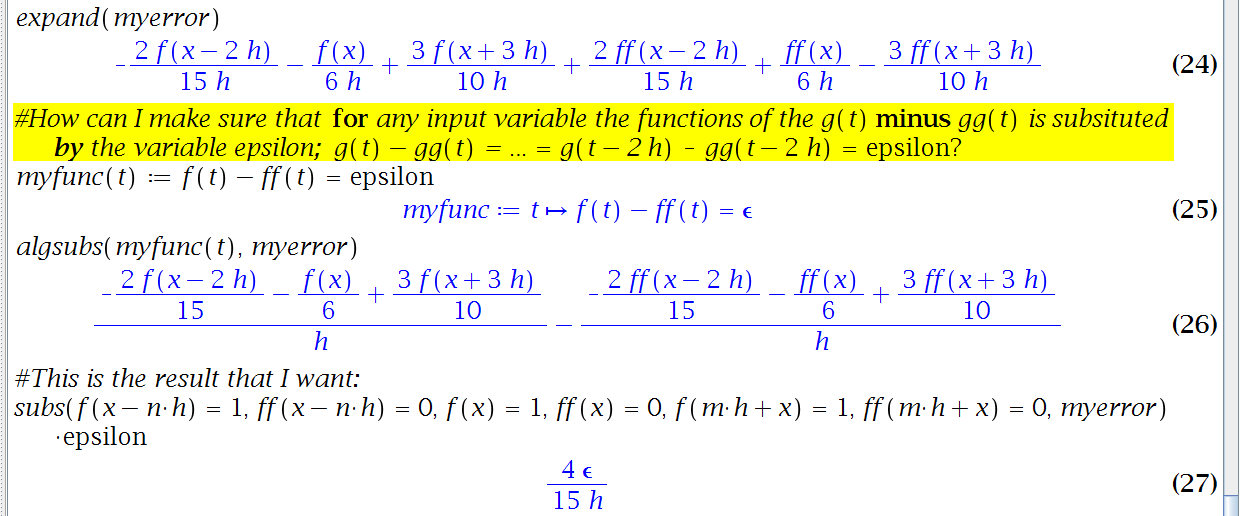expand(myerror);
2 f(x - 2 h)   f(x)   3 f(x + 3 h)   2 ff(x - 2 h)   ff(x)
- ------------ - ---- + ------------ + ------------- + -----
15 h       6 h        10 h           15 h         6 h

3 ff(x + 3 h)
- -------------
10 h

NULL;
myfunc := t -> f(t) - ff(t) = epsilon;
myfunc := proc (t) options operator, arrow, function_assign;

f(t)-ff(t) = epsilon end proc

algsubs(myfunc(t), myerror);
2               1        3
- -- f(x - 2 h) - - f(x) + -- f(x + 3 h)
15              6        10
----------------------------------------
h

2                1         3
- -- ff(x - 2 h) - - ff(x) + -- ff(x + 3 h)
15               6         10
- -------------------------------------------
h

NULL;
subs(f(-h*n + x) = 1, ff(-h*n + x) = 0, f(x) = 1, ff(x) = 0, f(h*m + x) = 1, ff(h*m + x) = 0, myerror)*epsilon;
4 epsilon
---------
15 h

## How do I change equations (in 2D-output) into defi...

How do I change equations from the 2D-output into definitions?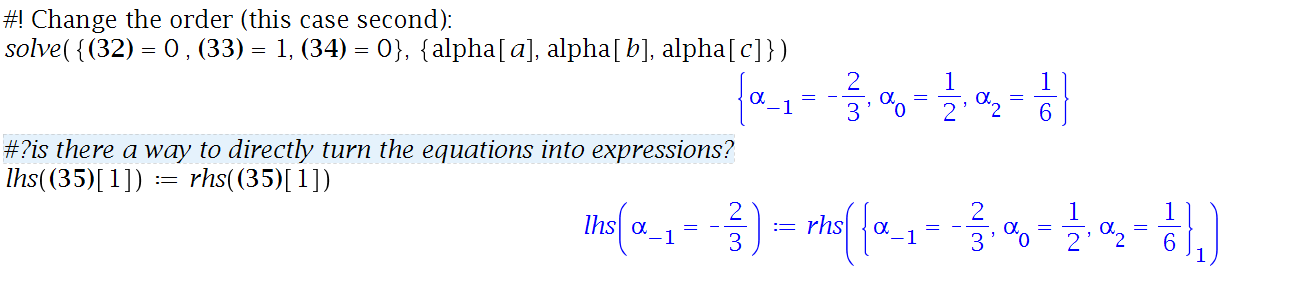#! Change the order (this case second):
solve({-alpha[-1] + 2*alpha = 1, 1/2*alpha[-1]*h + 2*alpha*h = 0, alpha[-1]/h + alpha/h + alpha/h = 0}, {alpha[a], alpha[b], alpha[c]});
/            -2             1             1\
{ alpha[-1] = --, alpha = -, alpha = - }
\            3              2             6/

NULL;
lhs({alpha[-1] = -2/3, alpha = 1/2, alpha = 1/6}) := rhs({alpha[-1] = -2/3, alpha = 1/2, alpha = 1/6});
/            -2\       / /            -2             1
lhs|alpha[-1] = --| := rhs|{ alpha[-1] = --, alpha = -,
\            3 /       \ \            3              2

1\    \
alpha = - }|
6/    /

## How can I make subs here replace this specific var...

Why am I not able to replace O(h^3), and in what other way can I achieve the result (I want to get the coefficients of the f(x), D(f)(x), and D^(2)(f)(x) variables).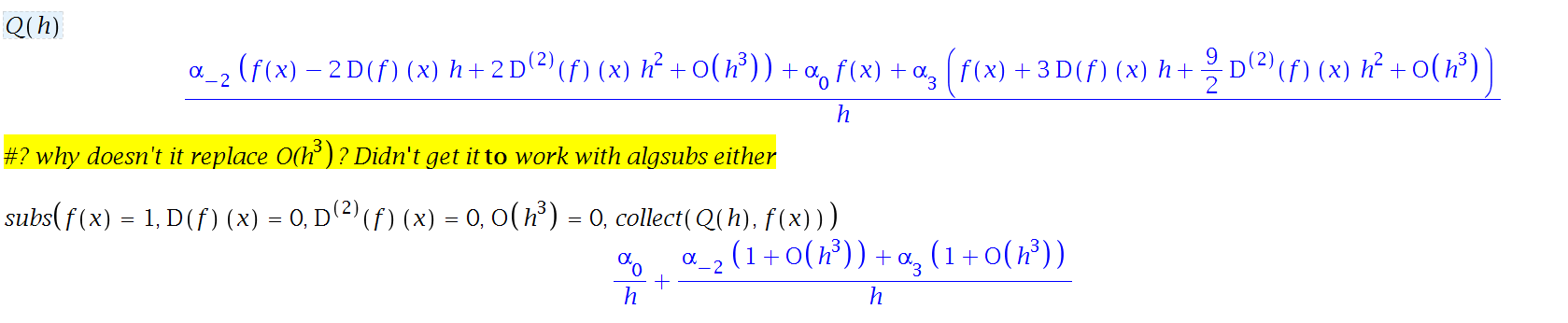Q(h);
1 /          /                                       2    / 3\\
- |alpha[-2] \f(x) - 2 D(f)(x) h + 2 @@(D, 2)(f)(x) h  + O\h //
h \

+ alpha f(x)

/                     9                 2    / 3\\\
+ alpha |f(x) + 3 D(f)(x) h + - @@(D, 2)(f)(x) h  + O\h /||
\                     2                          //

NULL;

subs(f(x) = 1, D(f)(x) = 0, (D@@2)(f)(x) = 0, O(h^3) = 0, collect(Q(h), f(x)));
/     / 3\\            /     / 3\\
alpha   alpha[-2] \1 + O\h // + alpha \1 + O\h //
-------- + --------------------------------------------
h                            h

## How do I combine greek letters and latin letters i...

How do I combine greek letters and latin letters in variable names? e.g. here I want to combine the greek letter Delta and the latin letter x to be used as one variable name: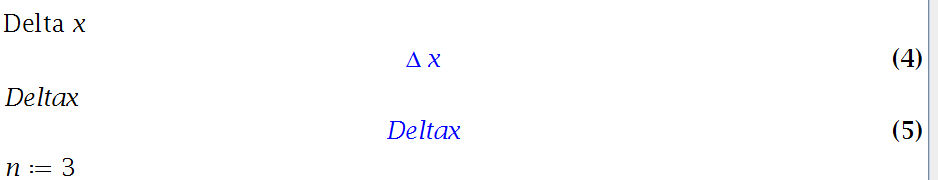Delta*x;
Delta x

Deltax;
Deltax

## How do I algebraicly expand an expression without ...

The original question (crossed through below) was too vague, so I tried to clarified it: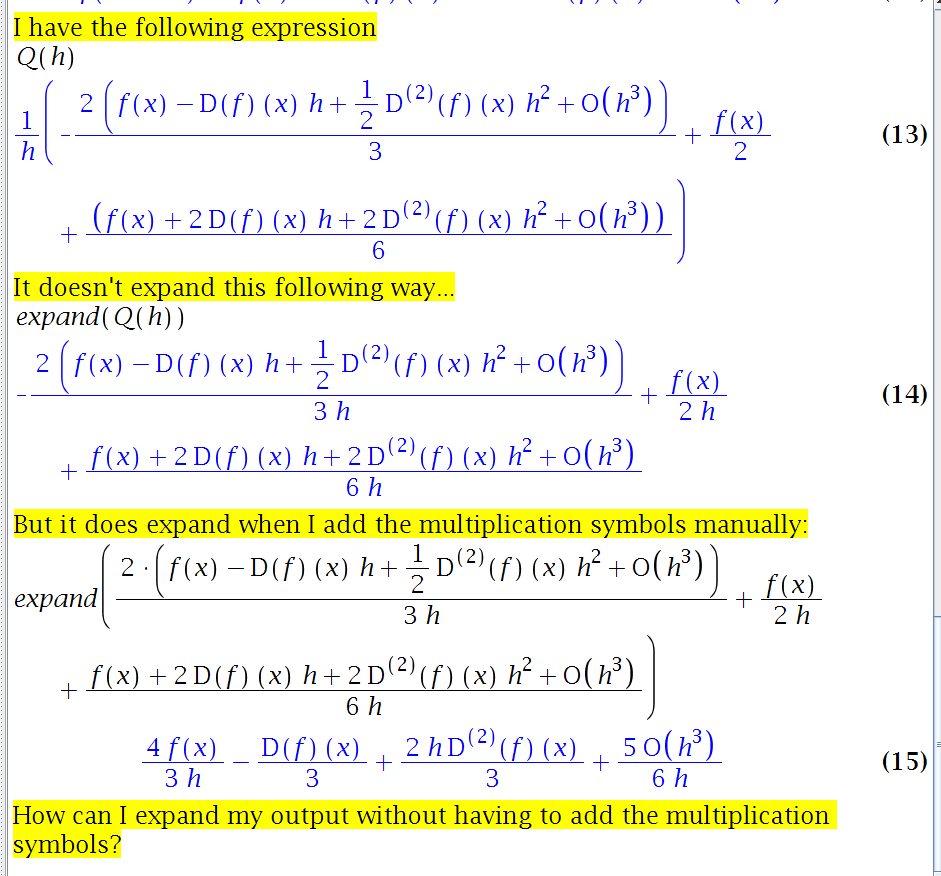I have the following expression
Q(h);
1 /  2                    1                 2    / 3\   1
- |- - f(x) - D(f)(x) h + - @@(D, 2)(f)(x) h  + O\h / + - f(x)
h \  3                    2                             2

1                                        2    / 3\\
+ - f(x) + 2 D(f)(x) h + 2 @@(D, 2)(f)(x) h  + O\h /|
6                                                 /

It doesn't expand this following way...
expand(Q(h));
/                   1                 2    / 3\\
2 |f(x) - D(f)(x) h + - @@(D, 2)(f)(x) h  + O\h /|
\                   2                          /   f(x)
- -------------------------------------------------- + ----
3 h                           2 h

2    / 3\
f(x) + 2 D(f)(x) h + 2 @@(D, 2)(f)(x) h  + O\h /
+ ------------------------------------------------
6 h

But it does expand when I add the multiplication symbols manually:
expand(2*(f(x) - D(f)(x)*h + 1/2*(D@@2)(f)(x)*h^2 + O(h^3))/(3*h) + f(x)/(2*h) + (f(x) + 2*D(f)(x)*h + 2*(D@@2)(f)(x)*h^2 + O(h^3))/(6*h));
/ 3\
4 f(x)   1           2                    5 O\h /
------ - - D(f)(x) + - h @@(D, 2)(f)(x) + -------
3 h     3           3                      6 h

How can I expand my output without having to add the multiplication symbols?

## why assuming works, but assume does not?...

I found there is assume=[...] syntax. So I changed my code to use it, instead of .... assuming

Now I find that some tests fail.  Should assuming and assume not give same result?

```restart;
sol :=y(x)^(1/2) = -1+2*exp(x):
ode:=diff(y(x),x)-2*y(x) = 2*y(x)^(1/2):

odetest(sol,ode) assuming x::real, x>0```

gives 0

but

`odetest(sol,ode,assume=[x::real, x >0])`

does not give zero. Please see screen shot below.

Reason I changed, since I started saving assumptions to use in a list. So it was easier to use assume=[....] than assuming ...., since assuming wants expression sequence in front of it and I did not know how to convert the list of assumptions I have collected to an expression sequence on the fly to use assuming, otherwise I would not have changed the code.

But my question is: Should both give same result? Why is the result different?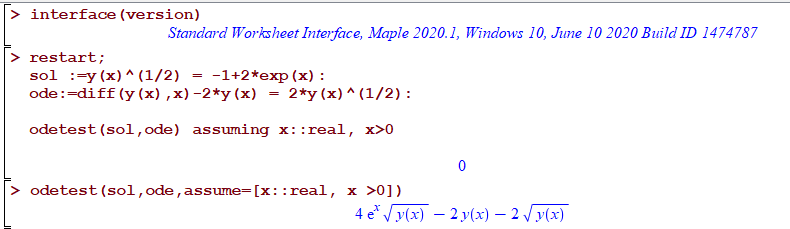Help says

The assume routine sets variable properties and relationships between variables. Similar functionality is provided by the assuming command.

I am probably not using assume= correctly.   I thought I could use the same thing I had on assuming, just put it in a list.

## does mserver.exe remember information about sessi...

This happens each time I run a long loop.  (2,500 iterations, which takes about 3 hrs to complete)

Maple always hangs (it does not time out on odetest() ). But my question is not about this (as this is something I have to deal with for long time now and mentioned it before many times. May be one day Maplesoft will fix this).

But I noticed this also.  When Maple hangs, (and it always hangs at least once during this loop), I then click on the button "interrupt the current operation". This does stop the hangs.

Next I do a restart and starts the loop from the loop counter where it hanged in order to continue.

But It still hangs at that same iteration. I repeate this again, and it still hangs.

Now I close Maple altogether, start Maple again, open same worksheet, and repeat from the same iteration again from where it was at before, now it does not hang.

This tells me that restart and "interrupt the current operation" do not clean everything as expected. Else why only restarting Maple makes it work?

It means mserver.exe (separate process from the frontend) still is caching something related, and that is why it hangs at that iteration.

I can reproduce this each time I run the whole loop from the start.

I can't make a minimal example, since I have no idea where it hangs and why. And it is related to running a long loop.

I just know it hangs when doing odetest() with timeout which never timeout, and it seems random at what iteration it decides to hang.

But my question is really basic here: Does mserver.exe keep any information about the earlier user session/worksheet even after restart ? help says that restarts clear internal memory of the kernel.

Isn't mserver.exe  the Maple kernel? If so, then what could explain that only restarting Maple clears the hang? I am just looking for ideas that could explain this.

This type of problem is the most annoying thing about Maple for me.

Maple 2020.1 on windows 10.

## How to insert a section where I want in my documen...

Whoever designed Maple's user-interface thinks very differently from me. How can I insert a 2nd section into Chapter 1? There's no space between the end of Section 1 and the end of Chapter 1 for me to put the cursor, so where should I put the cursor, and what should I do?